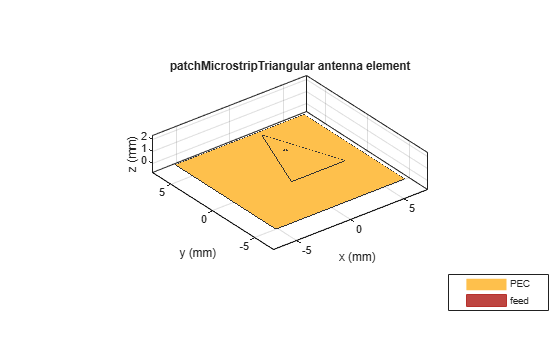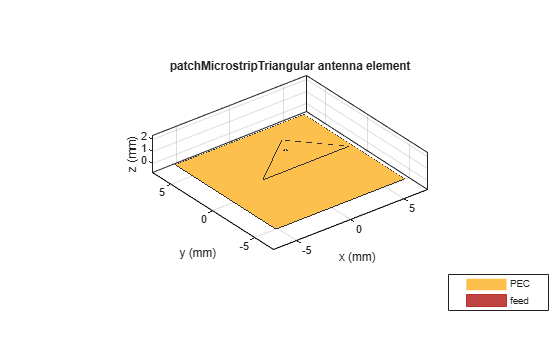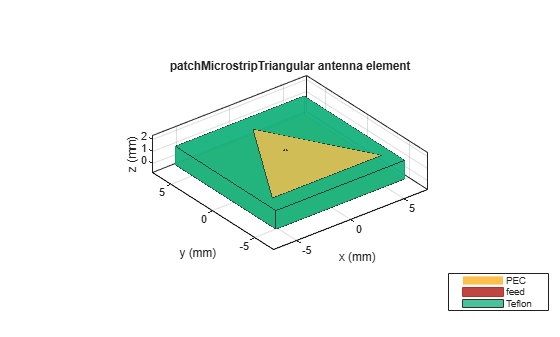# patchMicrostripTriangular

Create triangular microstrip patch antenna

## Description

Use the `patchMicrostripTriangular` object to create a triangular microstrip patch antenna. The default patch is centered at the origin. By default, the dimensions are chosen for an operating frequency of 15 GHz. If you use a Teflon substrate, the default operating frequency is at 12.5 GHz.## Creation

### Syntax

``trianglepatch = patchMicrostripTriangular``
``trianglepatch = patchMicrostripTriangular(Name,Value)``

### Description

example

````trianglepatch = patchMicrostripTriangular` creates a triangular microstrip patch antenna. ```

example

````trianglepatch = patchMicrostripTriangular(Name,Value)` sets properties using one or more name-value pairs. For example, `trianglepatch = patchMicrostripTriangular('Side',0.2)` creates a triangular microstrip patch with a side length of 0.2 m. Enclose each property name in quotes.```

## Properties

expand all

Side lengths of triangular patch, specified as a scalar in meters or a two or three-element vector with each element unit in meters.

• Equilateral triangle - `Side` property value is a scalar. All three sides of the triangle are equal.

• Isosceles triangle - `Side` property value is a two-element vector. The first value specifies the base of the triangle along the x-axis. The second value specifies the other two sides of the triangle.

• Scalene triangle - `Side` property value is a three-element vector. The first value specifies the base of the triangle along the x-axis. The remaining two values specify the other two sides of the triangle.

Example: `'Side',0.2`

Example: `trianglepatch.Side = [0.2,0.3,0.4]` where the first value is the base of the scalene triangle along the x-axis.

Data Types: `double`

Patch height above ground along z-axis, specified as a scalar in meters.

Example: `'Height',0.2`

Example: `trianglepatch.Height = 0.002`

Data Types: `double`

Ground plane length along x-axis, specified as a scalar in meters.

Example: `'GroundPlaneLength',120e-3`

Example: ```trianglepatch.GroundPlaneLength = 120e-3```

Data Types: `double`

Ground plane width along y-axis, specified as a scalar in meters.

Example: `'GroundPlaneWidth',120e-3`

Example: ```trianglepatch.GroundPlaneWidth = 120e-3```

Data Types: `double`

Signed distance of patch from origin, specified as a two-element real vector with each element unit in meters. Use this property to adjust the location of the patch relative to the ground plane. Distances are measured along the length and width of the ground plane.

Example: `'PatchCenterOffset',[0.01 0.01]`

Example: ```trianglepatch.PatchCenterOffset = [0.01 0.01]```

Data Types: `double`

Signed distance of feed from origin, specified as a two-element real vector with each element unit in meters. Use this property to adjust the location of the feedpoint relative to the ground plane and patch. Distances are measured along the length and width of the ground plane.

Example: `'FeedOffset',[0.01 0.01]`

Example: ```trianglepatch.FeedOffset = [0.01 0.01]```

Data Types: `double`

Feed diameter, specified as a scalar in meters.

Example: `'FeedDiameter',0.0600`

Example: ```trianglepatch.FeedDiameter = 0.0600```

Data Types: `double`

Type of the metal used as a conductor, specified as a metal material object. You can choose any metal from the `MetalCatalog` or specify a metal of your choice. For more information, see `metal`. For more information on metal conductor meshing, see Meshing.

Example: ```m = metal('Copper'); 'Conductor',m```

Example: ```m = metal('Copper'); ant.Conductor = m```

Type of dielectric material used as a substrate, specified as a dielectric material object. You can choose any material from the `DielectricCatalog` or use your own dielectric material. For more information, see `dielectric`. For more information on dielectric substrate meshing, see Meshing.

Note

The substrate dimensions must be lesser than the ground plane dimensions.

Example: `d = dielectric('FR4'); 'Substrate',d`

Example: `d = dielectric('FR4'); ant.Substrate = d`

Lumped elements added to the antenna feed, specified as a `lumpedElement` object. You can add a load anywhere on the surface of the antenna. By default, the load is at the feed. For more information, see `lumpedElement`.

Example: `'Load',lumpedElement`, where `lumpedElement` is load added to the antenna feed.

Example: `ant.Load = lumpedElement('Impedance',75)`

Tilt angle of the antenna, specified as a scalar or vector with each element unit in degrees. For more information, see Rotate Antennas and Arrays.

Example: `'Tilt',90`

Example: `ant.Tilt = 90`

Example: `'Tilt',[90 90]`,`'TiltAxis',[0 1 0;0 1 1]` tilts the antenna at 90 degrees about the two axes defined by the vectors.

Note

The `wireStack` antenna object only accepts the dot method to change its properties.

Data Types: `double`

Tilt axis of the antenna, specified as:

• Three-element vector of Cartesian coordinates in meters. In this case, each coordinate in the vector starts at the origin and lies along the specified points on the X-, Y-, and Z-axes.

• Two points in space, each specified as three-element vectors of Cartesian coordinates. In this case, the antenna rotates around the line joining the two points in space.

• A string input describing simple rotations around one of the principal axes, 'X', 'Y', or 'Z'.

Example: `'TiltAxis',[0 1 0]`

Example: `'TiltAxis',[0 0 0;0 1 0]`

Example: `ant.TiltAxis = 'Z'`

Note

The `wireStack` antenna object only accepts the dot method to change its properties.

Data Types: `double`

## Object Functions

 `show` Display antenna or array structure; display shape as filled patch `axialRatio` Axial ratio of antenna `beamwidth` Beamwidth of antenna `charge` Charge distribution on metal or dielectric antenna or array surface `current` Current distribution on metal or dielectric antenna or array surface `design` Design prototype antenna or arrays for resonance around specified frequency `efficiency` Radiation efficiency of antenna `EHfields` Electric and magnetic fields of antennas; Embedded electric and magnetic fields of antenna element in arrays `impedance` Input impedance of antenna; scan impedance of array `mesh` Mesh properties of metal or dielectric antenna or array structure `meshconfig` Change mesh mode of antenna structure `optimize` Optimize antenna or array using SADEA optimizer `pattern` Radiation pattern and phase of antenna or array; Embedded pattern of antenna element in array `patternAzimuth` Azimuth pattern of antenna or array `patternElevation` Elevation pattern of antenna or array `rcs` Calculate and plot radar cross section (RCS) of platform, antenna, or array `returnLoss` Return loss of antenna; scan return loss of array `sparameters` Calculate S-parameter for antenna and antenna array objects `vswr` Voltage standing wave ratio of antenna

## Examples

collapse all

Create and view a default triangular microstrip patch.

`p = patchMicrostripTriangular`
```p = patchMicrostripTriangular with properties: Side: 0.0102 Height: 0.0016 Substrate: [1x1 dielectric] GroundPlaneLength: 0.0120 GroundPlaneWidth: 0.0120 PatchCenterOffset: [0 0] FeedOffset: [0 5.4173e-04] FeedDiameter: 2.5000e-04 Conductor: [1x1 metal] Tilt: 0 TiltAxis: [1 0 0] Load: [1x1 lumpedElement] ```
`show(p)`Plot the radiation pattern at 15 GHz.

`pattern(p,15e9)`Create different types of triangles to use in the patch.

Equilateral Triangle

Create an equilateral triangle patch of side 7.2 mm.

```ant = patchMicrostripTriangular('Side',7.2e-3); show(ant);```Isosceles Triangle

Create an isosceles triangular patch antenna with sides using the following dimensions: 5 mm and 7.2 mm.

```ant = patchMicrostripTriangular('Side',[5e-3,7.2e-3]); show(ant);```In the above figure, you will see that the first value of the side is chosen as the base of the triangle.

Scalene Triangle

Create a scalene triangular patch antenna with side using the following dimensions: 8 mm, 5 mm, and 4 mm.

```ant = patchMicrostripTriangular('Side',[8e-3, 6e-3, 5e-3]); show(ant);```In the above figure, you will see that the first value of the side is chosen as the base of the triangle.

Create and view a triangular microstrip patch using Teflon substrate.

```d = dielectric('Teflon'); p = patchMicrostripTriangular('Substrate',d); show(p)```Plot the radiation pattern of the antenna.

`pattern(p,12.5e6)`## Version History

Introduced in R2018a